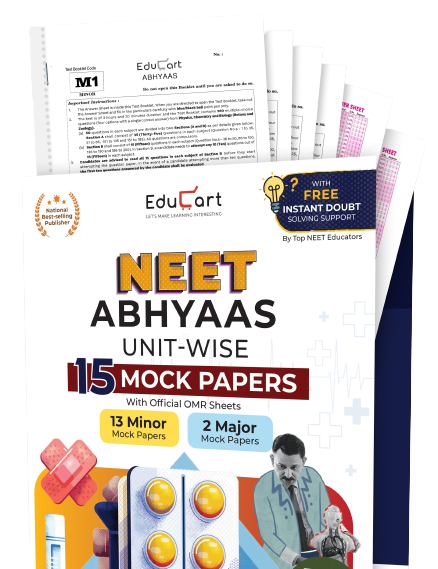# NCERT Books Class 11 MathematicsNCERT Books play the most important role in Class 9 to 12 exam preparation. Whether the students appear for standard unit tests or final board exams, it is important to follow NCERT books according to the CBSE-prescribed syllabus and paper pattern.

Class 11 Mathematics Complete Book

Class 11 Mathematics Chapters

Chapter 1 Sets
Chapter 2 Relations and Functions
Chapter 3 Trigonometric Functions
Chapter 4 Principle of Mathematic Induction
Chapter 5 Complex Numbers and Quadratic Equations
Chapter 6 Linear Inequalities
Chapter 7 Permutations and Combinations
Chapter 8 Binomial Theorem
Chapter 9 Sequence and Series
Chapter 10 Straight Lines
Chapter 11 Conic Sections
Chapter 12 Introduction to Three Dimensional Geometry
Chapter 13 Limits and Derivatives
Chapter 14 Mathematical Reasoning
Chapter 15 Statistics
Chapter 16 Probability